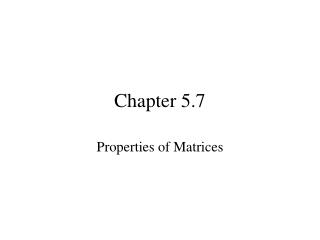Download PresentationChapter 5.7

# Chapter 5.7

Download Presentation## Chapter 5.7

- - - - - - - - - - - - - - - - - - - - - - - - - - - E N D - - - - - - - - - - - - - - - - - - - - - - - - - - -
##### Presentation Transcript

1. Chapter 5.7 Properties of Matrices

2. Basic Definitions It is necessary to use capital letters to name matrices. Also, subscript notation is often used to name element of a matrix, as in the following Matrix A.

3. With this notation, the first row, first column element is a11 (read “a-sub-one-one”); The second row, third column element is a23 and in general the i th row, j thcolumn element is aij

4. Certain matrices have special names: because the number of rows is equal to the number of columns. A matrix with just one row is a row matrix, and a matrix with just one column is a column matrix.

5. Two matrices are equal if they are the same size and if the corresponding element, position by position are equal. Using this definition, the matrices are not equal (even though they contain the same elements and are the same size), since the corresponding elements differ.

6. Find the values of the variables for which each statement is true, if possible.

7. Find the values of the variables for which each statement is true, if possible.

8. Adding Matrices Addition of matrices is defined as follows. It can be shown that matrix addition satisfies the commutative, associative, closure, identity, and inverse properties.

9. Find the sum if possible

10. Find the sum if possible

11. Find the sum if possible

12. Special Matrices A matrix containing only zero elements is called a zero matrix. A zero matrix can be written with any size.

13. By the additive inverse property, each real number has an additive inverse: if a is a real number, then there is a real number –a such that a + (-a) = 0 and –a + a = 0

14. Given matrix A, there is a matrix –A such that A + -A = 0 The matrix –A has as elements the additive inverses of the elements of A.

15. For example, if

16. For example, if

17. Matrix –A is called the additive inverse, or negative, of matrix A. Every matrix has an additive inverse Subtracting Matrices The real number b is subtracted from the real number a, written a – b, by adding a and the additive inverse of b. That is, a – b = a + (-b)

18. In practice, the difference of two matrices of the same size is found by subtracting corresponding elements.

19. Find the difference if possible

20. Find the difference if possible

21. Find the difference if possible

22. Multiplying Matrices In work with matrices, a real number is called a scalar to distinguish it from a matix. The product of a scalar K and a matrix X is the matrix kX, each of whose elements is k times the corresponding element of X.

23. Find the product

24. Find the product

25. We have seen how to multiply a real number (scalar) and a matrix. To find the product of two matrices, such as

26. first locate row 1 of A

27. first locate row 1 of A and column 1 of B,

28. Multiply corresponding elements, and find the sum of the products. • (-3)(-6) + (4) (2) + (2)(3) • + 8 + 6 = 32

29. The result is the first element for row 1, column 1 in the product matrix. • (-3)(-6) + (4) (2) + (2)(3) • + 8 + 6 = 32

30. Now use row 1 of A and column 2 of B to determine the element in row 1 and column 2 of the product matrix.

31. Now use row 1 of A and column 2 of B to determine the element in row 1 and column 2 of the product matrix. (-3)(4) + (4) (3) + (2)(-2) -12 + 12 + -4 = -4

32. Now use row 2 of A and column 1 of B to determine the element in row 2 and column 1 of the product matrix.

33. Now use row 2 of A and column 1 of B to determine the element in row 2 and column 1 of the product matrix. (5)(-6) + (0) (2) + (4)(3) -30 + 0 + 12 = -18

34. Now use row 2 of A and column 2 of B to determine the element in row 2 and column 2 of the product matrix.

35. Now use row 2 of A and column 2 of B to determine the element in row 2 and column 2 of the product matrix. (5)(4) + (0) (3) + (4)(-2) 20 + 0 + -8 = 12

36. The product matrix can now be written.

37. same size Can the product AB be calculated? The following diagram shows that AB can be calculated, because the number of columns of A is equal to the number of rows of B.

38. same size If AB can be calculated, how big is it? size of AB = 3 x 4

39. If BA can be calculated? BA cannot be calculated? different size

40. Find the product

41. Find the product

42. Find the product

43. Find the product

44. Find the product

45. Find the product

46. Find the product

47. Find the product